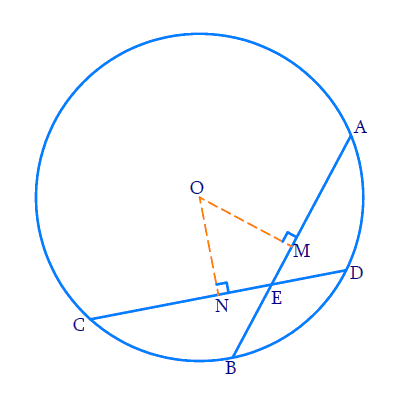In the verge of coronavirus pandemic, we are providing FREE access to our entire Online Curriculum to ensure Learning Doesn't STOP!

# Ex.10.4 Q2 Circles Solution - NCERT Maths Class 9

Go back to  'Ex.10.4'

## Question

If two equal chords of a circle intersect within the circle, prove that the segments of one chord are equal to corresponding segments of the other chord.

Video Solution
Circles
Ex 10.4 | Question 2

## Text Solution

What is known?

Chords are equal. Chords intersect at a point within the circle.

What is unknown?

Proof of corresponding segments of equal chords are equal.

Reasoning:

Equal chords are equidistant from the centre. Using this and Right angled-Hypotenuse-Side (RHS) criteria and Corresponding parts of congruent triangles (CPCT) we prove the statement.

Steps:Let $${AB}$$ and $${CD}$$ be the $$2$$ equal chords. $${AB = CD.}$$

Let the chords intersect at point $${E}$$. Join $${OE.}$$

To prove \begin{align} {AE = CE \, {\rm{and}} \, BE = DE.} \end{align}

Draw perpendiculars from the center to the chords. Perpendicular bisects the chord $${AB}$$ at $${M}$$ and $${CD}$$ at $${N.}$$

\begin{align} {AM = MB = CN = DN ……(1)} \end{align}

\begin{align} {\rm{In}} \;\Delta {OME}\; {\rm{and}} \;\Delta {ONE}\end{align}

\begin{align}\angle {M}&=\angle {N}=90^{\circ}\\ {OE}&={OE}\\{OM}&={ON} \quad\\\end{align}

Equal chords are equidistant from the centre.

By RHS criteria, $$\Delta {OME}$$ and $$\Delta {ONE}$$ are congruent.

So by $$\text{CPCT}, {ME}={NE} \ldots \ldots(2)$$

We know that: $${CE}={CN}+{NE}$$  and

$${AE}={AM}+{ME}$$

From ($$1$$) and ($$2$$), it is evident $${CE = AE}$$

$${DE}={CD}-{CE}$$ and

$${BE}={AB}-{AE}$$

$${AB}$$ and $${CD}$$ are equal, $${CE}$$ and $${AE}$$ are equal. So $${DE}$$ and $${BE}$$ are also equal.

It is proved corresponding segments of equal chords are equal.

Video Solution
Circles
Ex 10.4 | Question 2

Learn from the best math teachers and top your exams

• Live one on one classroom and doubt clearing
• Practice worksheets in and after class for conceptual clarity
• Personalized curriculum to keep up with school